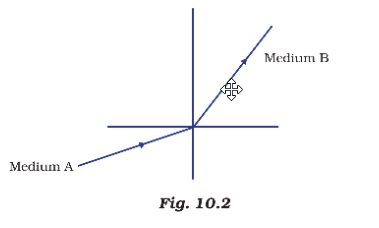MCQs from NCERT Exemplar

Class 10
Chapter 10 Class 10 - Light - Reflection and Refraction (Term 1)

## A light ray enters from medium A to medium B as shown in Figure 10.2. The refractive index of mediumB relative to A will be## (d) zero

Relative refractive index = N 2 /N 1

In this case, Relative refractive index, ղ = N b /N a

• Going from Medium A to B, light ray bends towards the normal
• When an incident ray passes from rarer to denser medium , it bends toward the normal.
• Hence, Medium B is denser than Medium A
• So, we can say that N b > N a .
• Thus, Relative refractive index, ղ = N b /N a > 1

So, the correct answer is (a)